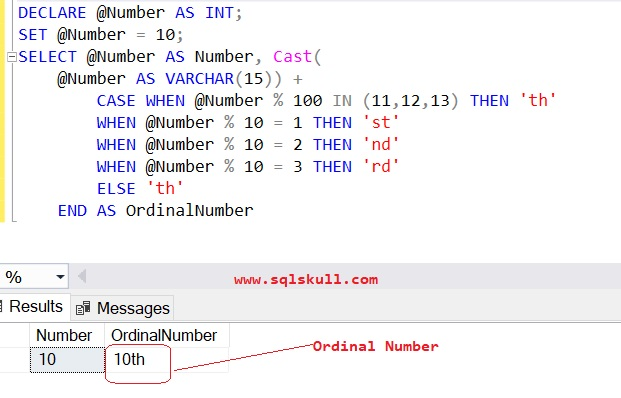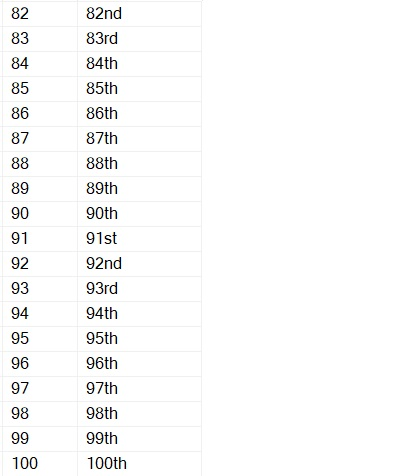Home » How to get Ordinal Number in SQL

# How to get Ordinal Number in SQLIf any number that is written like as 1st, 2nd, 3rd and so on.. is called an ordinal number, and it is read as 1st( first), 2nd(second), 3rd(third), 4th fourth respectively.

By adding a letter st, nd, rd, and th in the end of any number, makes that number an ordinal number.

Lets create a T-SQL that returns an ordinal number for any specified number.

Following T-SQL query, returns an ordinal number for specified number.

```DECLARE @Number AS INT;
SET @Number = 10;
SELECT @Number AS Number, Cast(
@Number AS VARCHAR(15)) +
CASE WHEN @Number % 100 IN (11,12,13) THEN 'th'
WHEN @Number % 10 = 1 THEN 'st'
WHEN @Number % 10 = 2 THEN 'nd'
WHEN @Number % 10 = 3 THEN 'rd'
ELSE 'th'
END AS OrdinalNumber```You can see the ordinal number for 10 is 10th. Similarly you can get ordinal number of any number that you will specify to variable @Number.

Lets use the same logic with Recursive CTE, and create an ordinal number for 1 to 100 numbers.

```WITH CTE_OrdinalNumbers
AS
(
SELECT 1 AS N
UNION ALL
SELECT N + 1
FROM CTE_OrdinalNumbers
WHERE N < 100
)
SELECT N AS Number, CAST(N AS VARCHAR(15))
+
CASE
WHEN N % 100 IN (11,12,13) THEN 'th'
WHEN N % 10 = 1 THEN 'st'
WHEN N % 10 = 2 THEN 'nd'
WHEN N % 10 = 3 THEN 'rd'
ELSE 'th'
END AS OrdinalNumber
FROM CTE_OrdinalNumbers
OPTION (MAXRECURSION 0)```…………….| |………………You can see, an ordinal number for 1 to 100 are returned that is 1st to 100th which can be seen in column OrdinalNumber.